• 阅读章节：第二章：感知机
• 开始时间：2018-09-18
• 结束时间：2018-09-21
• 目标：读完第二章，掌握基本概念，产出一篇笔记
• 博客地址

• 感知机模型
• 感知机学习策略
• 感知机学习算法

## 感知机模型

• 输入空间：输入所有可能取值的集合
• 输出空间：输出所有可能取值的集合
• 特征空间：每个具体的输入是一个实例，由特征向量表示

• 输入空间（特征空间）：$\chi \subseteq \mathbb{R} ^n$
• 输出空间：$\gamma = \{+1,-1 \}$

$$\displaystyle f( x) \ =\ sign( w\cdot x+b)$$

• $sign$: 符号函数
• $w$: 权值（weight）或权值向量（weight vector）
• $b$: 偏置（bias）

$$w\cdot x + b =0$$

• $w$: 超平面的法向量
• $b$: 超平面的截距

## 感知机学习策略

### 数据集的线性可分性

$$T = {(x_1,y_1),(x_2,y_2),…,(x_n,y_n)}$$

### 感知机学习策略

$$\frac{1}{||w||}|w\cdot x_0+b|$$

$$-\frac{1}{||w||}\sum_{x_i\in M} y_i(w\cdot x_i + b)$$

$$L(w,b) =-\sum_{x_i\in M} y_i(w\cdot x_i + b)$$

## 感知机学习算法

### 感知机学习算法的原始形式

$$\min_{w,b} L(w,b) =-\sum_{x_i\in M} y_i(w\cdot x_i + b)$$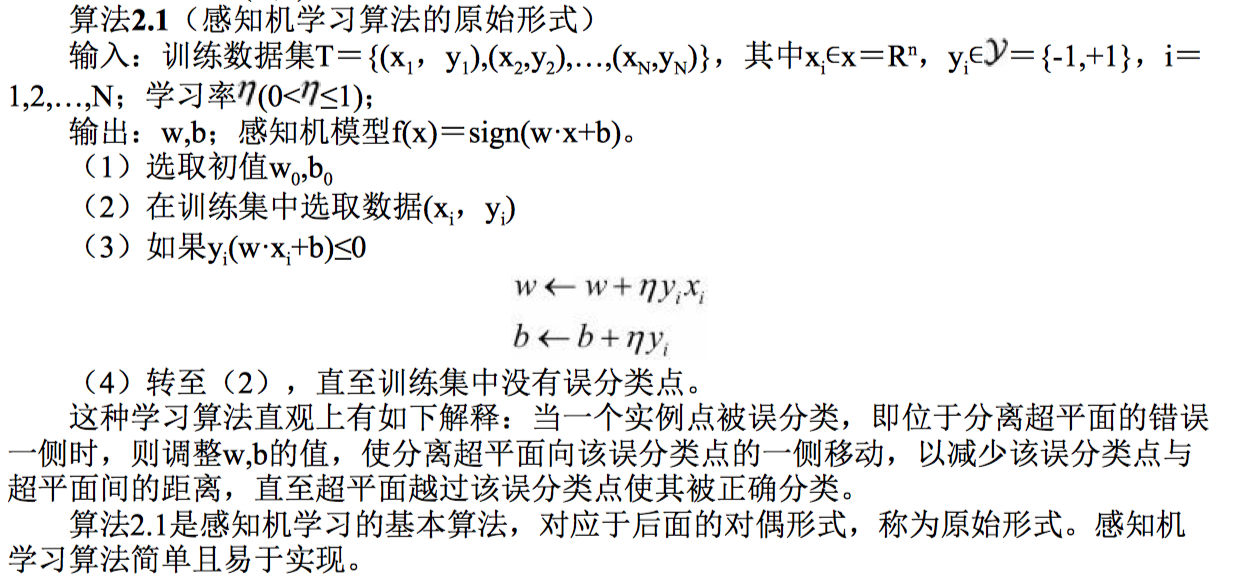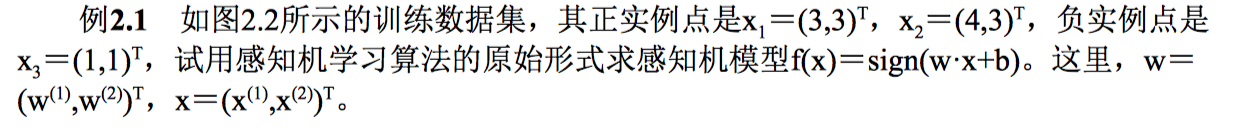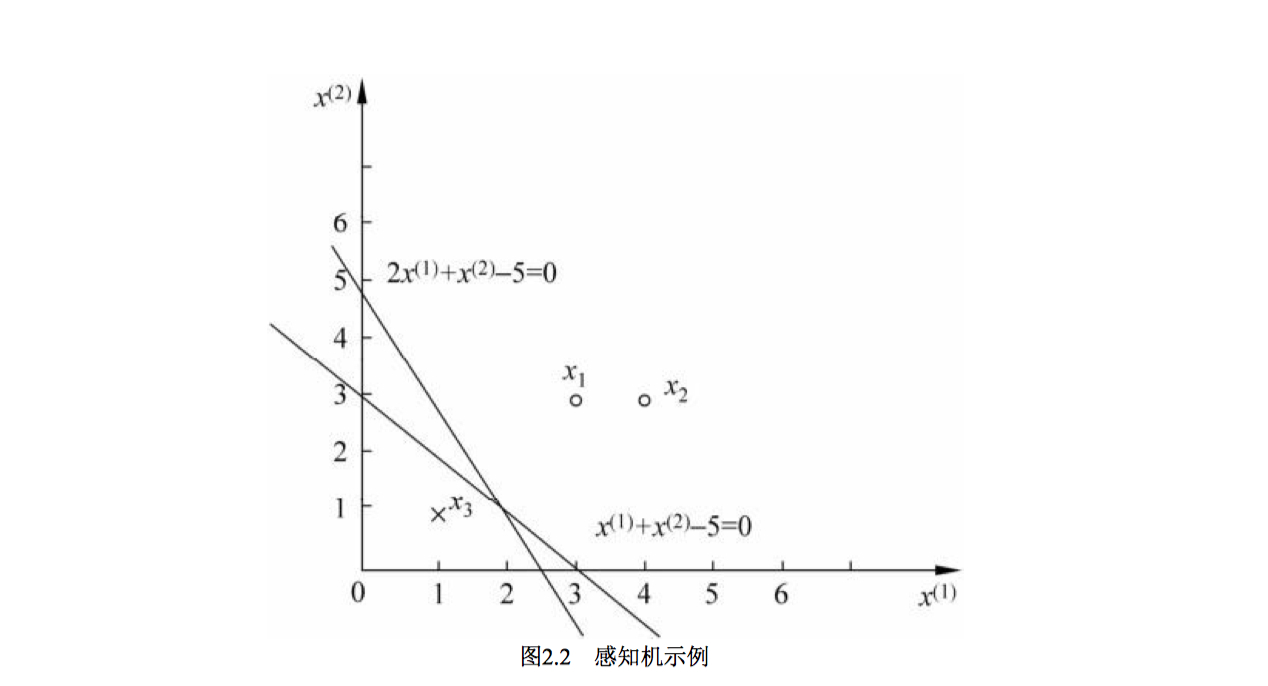1 2 3 4 5 6 7 8 9 10 11 12 13 14 15 16 17 18 19 20 21 22 23 24 25 26 27 28 29 30 31 32 33 34 35 36 37 38 39 40 41 42 43 44 45 46 47 48 49 50 51 52 53 54 55 56 57 58  #!/usr/bin/env python """ Created by howie.hu at 2018/9/20. """ import numpy as np class Perceptron: """ 李航老师统计学习方法第二章感知机例2.1代码实现 """ def __init__(self, input_nums=2): # 权重 已经确定只会有两个二进制输入 self.w = np.zeros(input_nums) # 偏置项 self.b = 0.0 def fit(self, input_vectors, labels, learn_nums=10, rate=1): """ 训练出合适的 w 和 b :param input_vectors: 样本训练数据集 :param labels: 标记值 :param learn_nums: 学习多少次 :param rate: 学习率 """ for i in range(learn_nums): for index, input_vector in enumerate(input_vectors): label = labels[index] delta = label * (sum(self.w * input_vector) + self.b) if delta <= 0: # 计算方法由梯度下降算法推导出来 self.w += label * input_vector * rate self.b += rate * label break print("最终结果：此时感知器权重为{0}，偏置项为{1}".format(self.w, self.b)) return self def predict(self, input_vector): """ 跃迁函数作为激活函数，感知器 :param input_vector: :return: """ if isinstance(input_vector, list): input_vector = np.array(input_vector) y = sum(self.w * input_vector) + self.b return 1 if y > 0 else -1 if __name__ == '__main__': input_vectors = np.array([[3, 3], [4, 3], [1, 1]]) labels = np.array([1, 1, -1]) p = Perceptron() model = p.fit(input_vectors, labels) print(model.predict([3, 3])) print(model.predict([4, 3])) print(model.predict([1, 1])) 

 1 2 3 4  最终结果：此时感知器权重为[ 1. 1.]，偏置项为-3.0 1 1 -1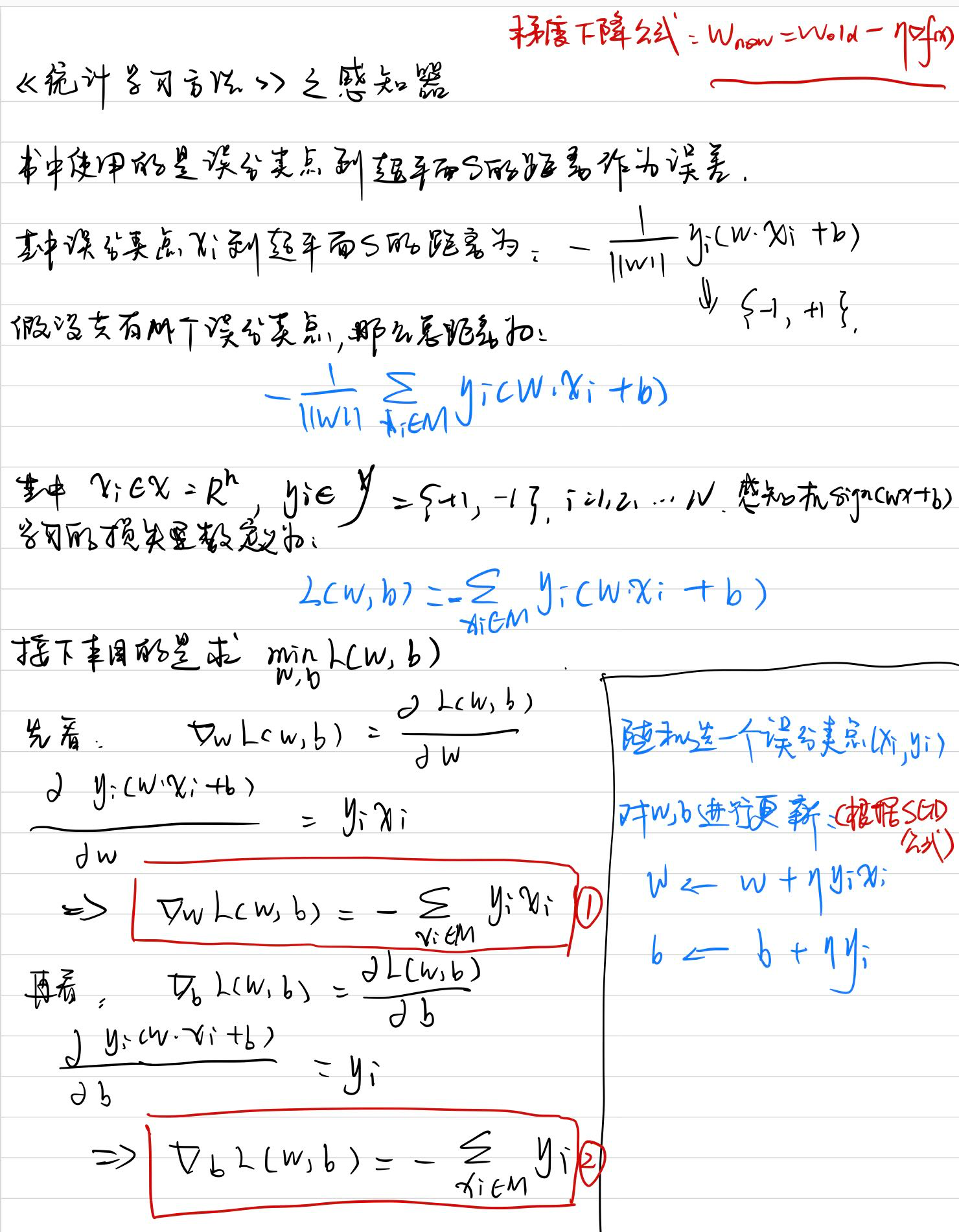### 算法的收敛性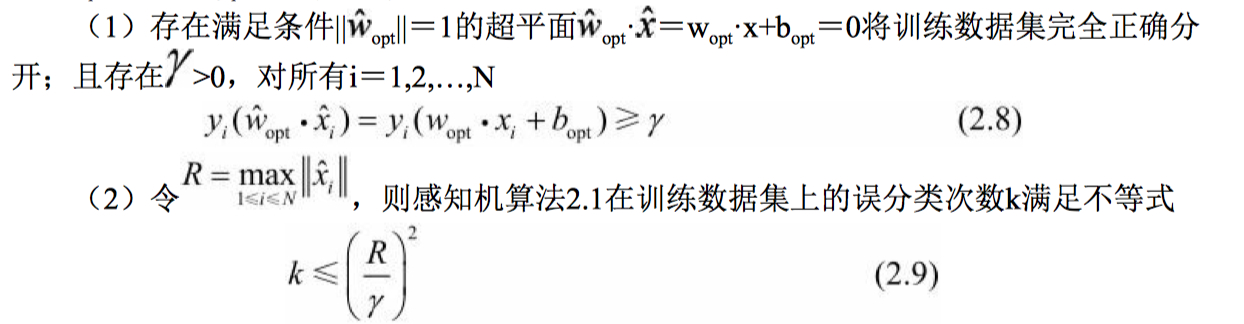### 感知机学习算法的对偶形式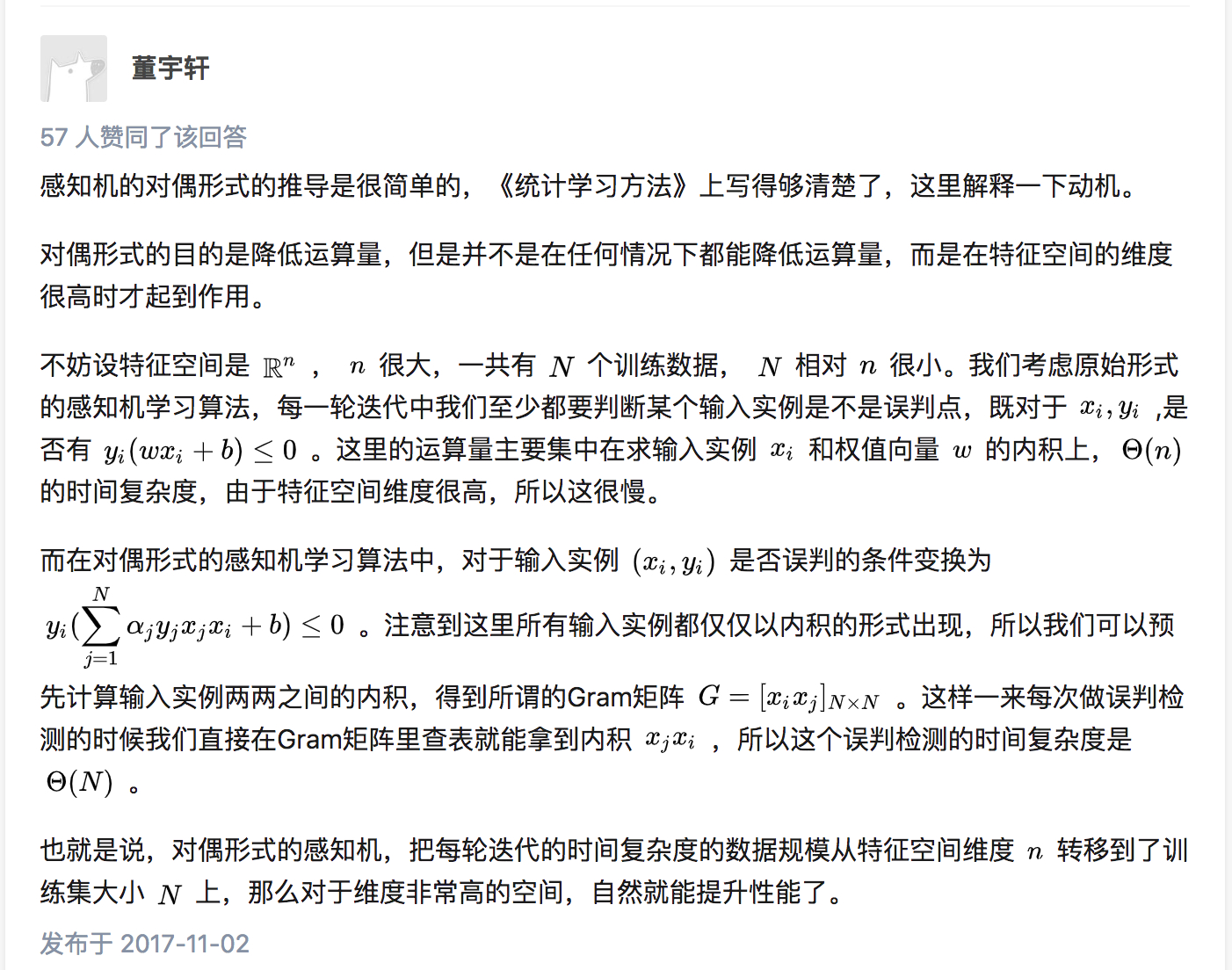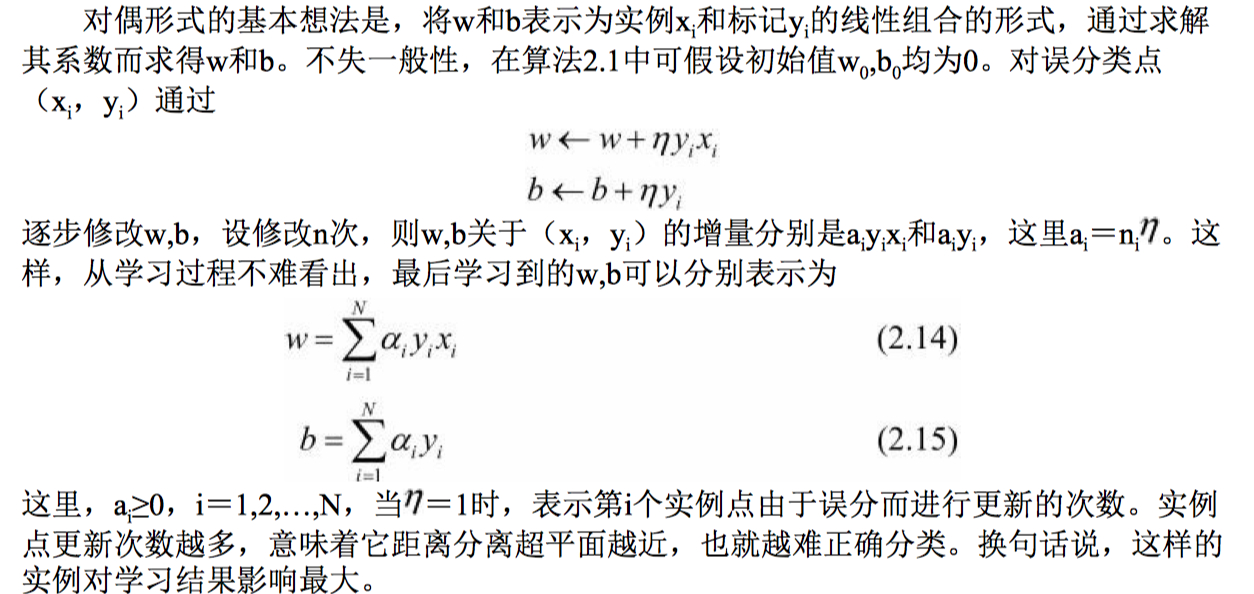1 2 3 4 5 6 7 8 9 10 11 12 13 14 15 16 17 18 19 20 21 22 23 24 25 26 27 28 29 30 31 32 33 34 35 36 37 38 39 40 41 42 43 44 45 46 47 48 49 50 51 52 53 54 55 56 57 58 59 60  #!/usr/bin/env python """ Created by howie.hu at 2018/9/21. """ import numpy as np class Perceptron: """ 李航老师统计学习方法第二章感知机例2.2对偶形式代码实现 """ def __init__(self, alpha_length=3): self.alpha = np.zeros(alpha_length) # 权重 self.w = np.zeros(2) # 偏置项 self.b = 0.0 def fit(self, input_vectors, labels, learn_nums=7): """ 训练出合适的 w 和 b :param input_vectors: 样本训练数据集 :param labels: 标记值 :param learn_nums: 学习多少次 """ gram = np.matmul(input_vectors, input_vectors.T) for i in range(learn_nums): for input_vector_index, input_vector in enumerate(input_vectors): label = labels[input_vector_index] delta = 0.0 for alpha_index, a in enumerate(self.alpha): delta += a * labels[alpha_index] * gram[input_vector_index][alpha_index] delta = label * delta + self.b if delta <= 0: self.alpha[input_vector_index] += 1 self.b += label break self.w = sum([j * input_vectors[i] * labels[i] for i, j in enumerate(self.alpha)]) print("最终结果：此时感知器权重为{0}，偏置项为{1}".format(self.w, self.b)) return self def predict(self, input_vector): if isinstance(input_vector, list): input_vector = np.array(input_vector) y = sum(self.w * input_vector) + self.b return 1 if y > 0 else -1 if __name__ == '__main__': input_vectors = np.array([[3, 3], [4, 3], [1, 1]]) labels = np.array([1, 1, -1]) p = Perceptron() model = p.fit(input_vectors, labels) print(model.predict([3, 3])) print(model.predict([4, 3])) print(model.predict([1, 1])) 
 1 2 3 4  最终结果：此时感知器权重为[ 1. 1.]，偏置项为-3.0 1 1 -1